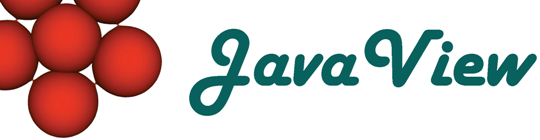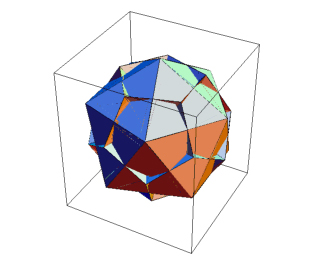Overview  Gallery  Introduction  Objects  Packages  Packages 3D  Basic Options  3D Options  Advanced

## 6. Working with 3D Options

### 3D Options / Box

#### Boxed

 The bounding box can be switched on or off. In:= b = Graphics3D[Stellate[Dodecahedron[]], Boxed -> False]; Show[b]In:= JavaView[b] Compare with Mathematica

#### BoxRatios

 BoxRatios is an option for Graphics3D and SurfaceGraphics which gives the ratios of side lengths for the bounding box of the three�dimensional picture. In:= br = Graphics3D[Stellate[Dodecahedron[]], BoxRatios -> {1., 2., 1.4}]; Show[br]In:= JavaView[br] Compare with Mathematica

#### FaceGrids

 FaceGrids is an option for three�dimensional graphics functions that specifies grid lines to draw on the faces of the bounding box. In:= fg = Graphics3D[Stellate[Dodecahedron[]], FaceGrids -> {{-1, 0, 0}, {1, 0, 0}}]; Show[fg]In:= JavaView[fg] Compare with Mathematica

#### AxesEdge

 AxesEdge is an option for three�dimensional graphics functions that specifies on which edges of the bounding box axes should be drawn. In:= ae = Graphics3D[Stellate[Dodecahedron[]], Axes -> True, AxesEdge -> {{-1, -1}, {-1, 1}, {1, 1}}]; Show[ae]In:= JavaView[ae] Compare with Mathematica

#### BoxStyle

 BoxStyle is an option for three�dimensional graphics functions which specifies how the bounding box should be rendered. In:= bs = Graphics3D[Stellate[Dodecahedron[]], BoxStyle -> {RGBColor[1, 0, 0], Dashing[{0.05, 0.05}]}]; Show[bs]In:= JavaView[bs] Compare with Mathematica

### 3D Options / Light

#### Lighting

 Lighting is a flag to disable the automatics lighting and use default coloring by height or apply a ColorFunction. In:= plf = Plot3D[Sin[xy], {x, 0, 3Pi/2}, {y, 0, 3Pi/2}, Lighting -> False]; Show[plf]In:= JavaView[plf] Compare with Mathematica

#### LightSources

 LightSources is an option forGraphics3D and related functions that specifies the properties of point light sources for simulated illumination. In:= ls = Graphics3D[Stellate[Dodecahedron[]], LightSources -> {{{1., 0., 0.}, RGBColor[1., 0.4, 0.4]}, {{-.15, .85, 0.}, RGBColor[0.4, 1., 0.4]}, {{-.15, -.85, 0.}, RGBColor[0.4, 0.4, 1.]}}]; Show[ls]In:= JavaView[ls] Compare with Mathematica

#### AmbientLight

 AmbientLight is an option for Graphics3D and related functions that gives the level of simulated ambient illumination in a three�dimensional picture. In:= al = Graphics3D[Stellate[Dodecahedron[]], AmbientLight -> RGBColor[0., 0.3, 0.]]; Show[al]In:= JavaView[al] Compare with Mathematica

 Shading is an option that specifies whether the surfaces should be shaded. In:= s = Graphics3D[ Stellate[Dodecahedron[]], Shading -> False]; Show[s]In:= JavaView[s] Compare with Mathematica

### 3D Options / Mesh

This options are shown with a SurfaceGraphics object. Mathematica scales the surface automatically to box ratios x : y : z = 1 : 1 : 0.4 and clips very high and low z-values. JavaView shows the surface with the correct z coordinates, without automatic clipping so the surface looks different.

#### Mesh

 Mesh is an option for SurfaceGraphics and DensityGraphics that specifies whether an explicit x�y mesh should be drawn. In:= nm = SurfaceGraphics[Table[Sin[x]*Exp[y], {x, -Pi, Pi, Pi/4.}, {y, -E, E, E/4.}], Mesh -> False]; Show[nm]In:= JavaView[nm] Compare with Mathematica

#### MeshStyle

 MeshStyle is an option forPlot3D,DensityPlot and related functions which specifies how mesh lines should be rendered. In:= ms = SurfaceGraphics[Table[Sin[x]*Exp[y], {x, -Pi, Pi, Pi/4.}, {y, -E, E, E/4.}], MeshStyle -> {Thickness[0.01], RGBColor[1., 0., 0.], Dashing[{0.02, 0.02}]}]; Show[ms]In:= JavaView[ms] Compare with Mathematica

#### MeshRange

 MeshRange is an option for ListPlot3D, SurfaceGraphics, ListContourPlot, ListDensityPlot and related functions which specifies the range of a and a coordinates that correspond to the array of a values given. In:= mr = SurfaceGraphics[Table[Sin[x]*Exp[y], {x, -Pi, Pi, Pi/4.}, {y, -E, E, E/4.}], MeshRange -> {{-Pi, Pi}, {-E, E}}, Axes -> True]; Show[mr]In:= JavaView[mr] Compare with Mathematica

#### ClipFill

 ClipFill is an option for SurfaceGraphics that specifies how clipped parts of the surface are to be drawn. In:= cf = SurfaceGraphics[Table[Sin[x]*Exp[y], {x, -Pi, Pi, Pi/4.}, {y, -E, E, E/4.}], ClipFill -> {GrayLevel[0.], GrayLevel[1.]}]; Show[cf]In:= JavaView[cf] Compare with Mathematica

See 3D Options / Light.

#### HiddenSurface

 HiddenSurface is an option for SurfaceGraphics which specifies whether faces are drawn. See also Shading. In:= hs = SurfaceGraphics[Table[Sin[x]*Exp[y], {x, -Pi, Pi, Pi/4.}, {y, -E, E, E/4.}], HiddenSurface -> False]; Show[hs]In:= JavaView[hs] Compare with Mathematica

### 3D Options / View

#### ViewPoint

 This option defines (in coordinates relative to the bounding box) the camera position. In:= vp = Graphics3D[Stellate[Dodecahedron[]], ViewPoint -> {3., 3., 1.}]; Show[vp]In:= JavaView[vp] Compare with Mathematica

#### ViewCenter

 The command ViewCenter modifies the interest of the camera. In:= vc = Graphics3D[Stellate[Dodecahedron[]], ViewCenter -> {1, 1, 1}]; Show[vc]In:= JavaView[vc] Compare with Mathematica

#### ViewVertical

 ViewVertical is an option for Graphics3D and SurfaceGraphics which specifies what direction in scaled coordinates should be vertical in the final image. In:= vv = Graphics3D[Stellate[Dodecahedron[]], ViewVertical -> {1., 1., 1.}]; Show[vv]In:= JavaView[vv] Compare with Mathematica

#### SphericalRegion

Shows no effect in JavaView. SphericalRegion is an option to scale the geometry inside the display such, that it could be rotated without exceeding the display size - this should be ever possible in JavaView.

#### PolygonIntersections

 PolygonIntersections is an option for Graphics3D which specifies whether intersecting polygons should be left unchanged.Note: because of the large size of the MGS file (1 MB) the right cell shows an image. Follow the comparison link to watch the applet. In:= pi = Show[Graphics3D[{Stellate[Dodecahedron[], -0.5], Icosahedron[]}, PolygonIntersections -> False]]; Show[pi, PlotRagne-> All]In:= JavaView[pi]Compare with Mathematica